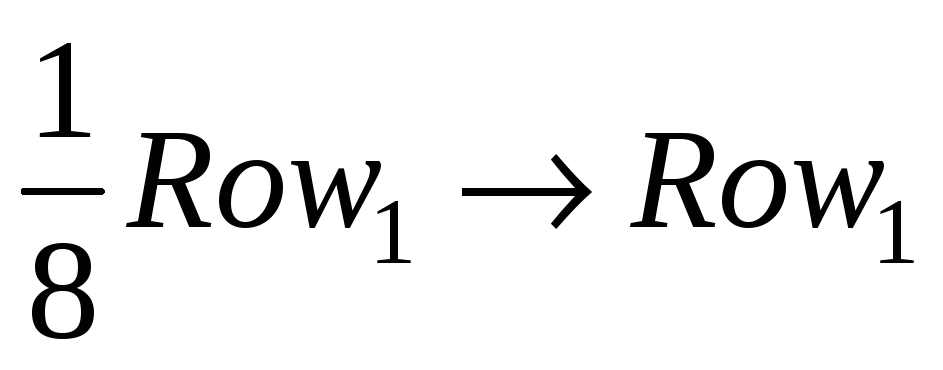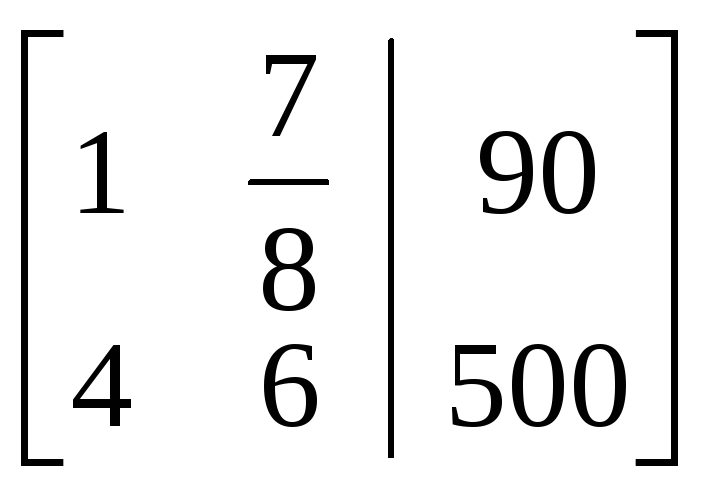# Section 4-2 Augmented Matrices

 Page 1/5 Date 02.05.2018 Size 0.52 Mb.
1   2   3   4   5
Section 4-2 Augmented Matrices
Example 1: Solve the following system of linear equations in two variables: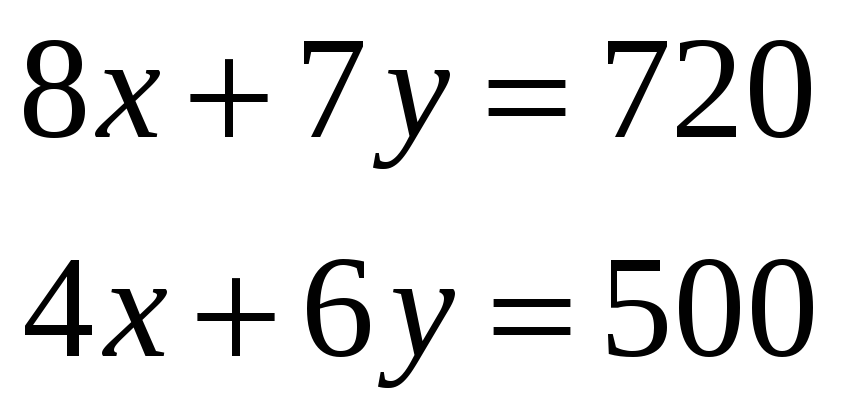This system can be represented as an augmented matrix where the first column contains the coefficients of x, the second column contains the coefficients of y, and the third column contains the constant terms. The first row represents the first equation and the second row represents the second equation: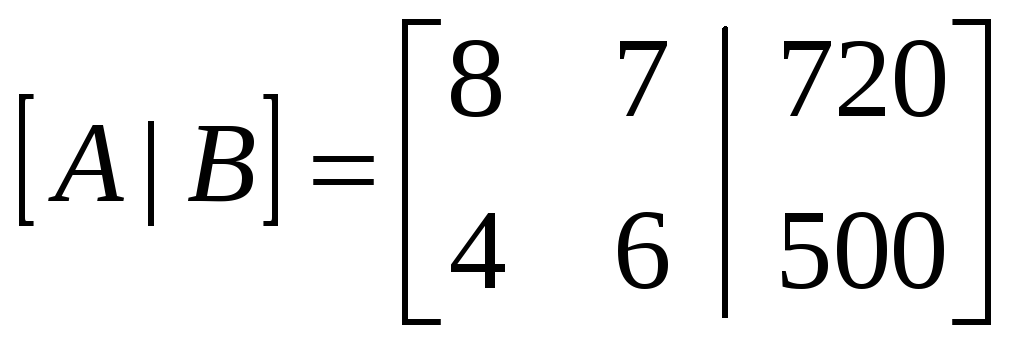We can solve this system of equations in a systematic fashion. In the illustration below, the arrow indicates that the expression on the left replaces the row indicated on the right.

 Start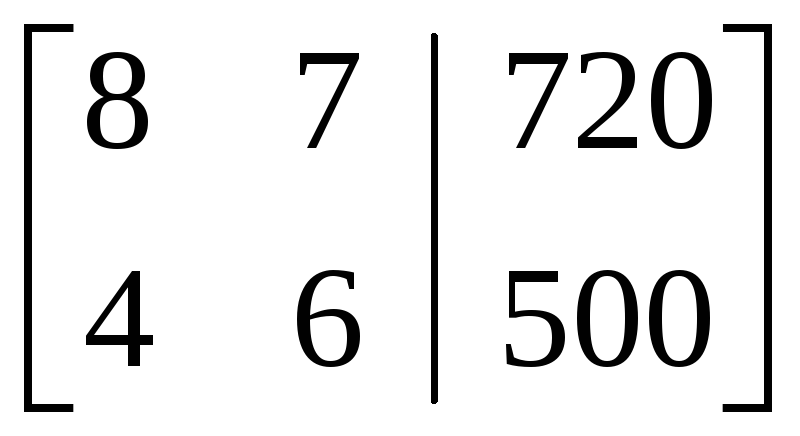Step 1. Set the coefficient of x in the first row to 1.